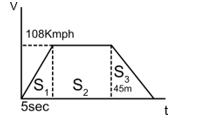Average Velocity
Question

# A metro train starts from rest and in 5 s achieves 108 km${\mathrm{h}}^{-1}$. After that it moves with constant velocity and comes to rest after travelling 45 m with uniform retardation. If total distance travelled is 390 m, find total time of travelling.

Easy
Solution

## Velocity time graph  is${\mathrm{S}}_{1}=\frac{1}{2}\mathrm{vt}=\frac{1}{2}\left(108×\frac{5}{18}\right)5=75\mathrm{m}\text{\hspace{0.17em}\hspace{0.17em}\hspace{0.17em}\hspace{0.17em}\hspace{0.17em}\hspace{0.17em}}{\mathrm{t}}_{1}=5\mathrm{sec}$${S}_{2}$ =390-75-45=270m${\text{t}}_{2}=\frac{270}{30}=9\mathrm{sec}$${\mathrm{s}}_{3}=\frac{1}{2}×30×{\mathrm{t}}_{3}=45$${\mathrm{t}}_{3}=\frac{45×2}{30}=3\mathrm{sec}$Total time = 9+5+3=17 sec

Get Instant Solutions